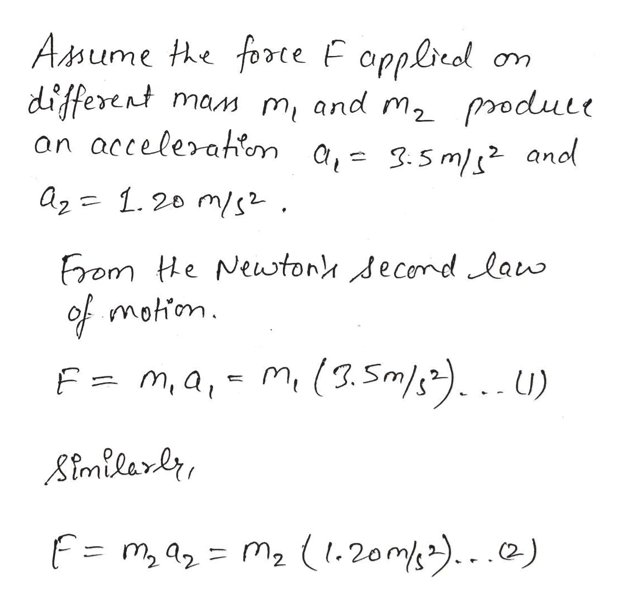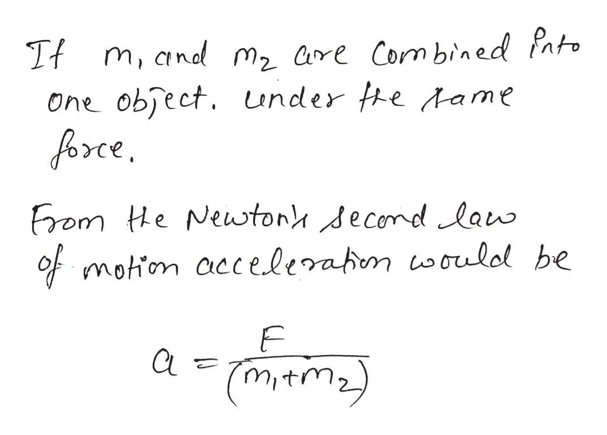A force applied to an object of mass m1 produces an acceleration of 3.50 m/s2. The same force applied to a second object of mass m2 produces an acceleration of 1.20 m/s2.If m1 and m2 are combined into one object, find its acceleration under the action of the force .

Question

A force applied to an object of mass m1 produces an acceleration of 3.50 m/s2. The same force applied to a second object of mass m2 produces an acceleration of 1.20 m/s2.

If m1 and m2 are combined into one object, find its acceleration under the action of the force .

Step 1help_outlineImage TranscriptioncloseAmume he forte F opplreol different an accelerahon a,= 3.5m/s and mam m and m2 produle az 1.20 m/s Erom He Newtony decomdlaw of motion F= m,a,- m(3.Sm).U) Aimilarer F= m, a= m2 (1.20m).) fullscreen
Step 2help_outlineImage TranscriptioncloseTf m, and m2 are Combin ed Pato One obfect. under fhe Aame orce From He Newwton decomdlaw of motion accelerabon woulol be tm2 fullscreen

Want to see the full answer?

See Solution

Want to see this answer and more?

Our solutions are written by experts, many with advanced degrees, and available 24/7

See Solution
Tagged in

Newtons Laws of Motion